In :
using LinearAlgebra
using Statistics
using SparseArrays
using MLDatasets
using PyPlot


## Test of Johnson-Lindenstrauss transform¶

In :
d = 100000;
n = 100;

Xs = randn(d,n); # the dataset

In :
epsilon = 0.1;
D = Int64(ceil(8 * log(n) / epsilon^2))

Out:
3685
In :
A = randn(D, d) / sqrt(D);

In :
# let's loook at this for a single example
norm(Xs[:,1] - Xs[:,2])

Out:
447.3594790493648
In :
norm(A*Xs[:,1] - A*Xs[:,2])

Out:
453.60422837944606
In :
norm(A*Xs[:,1] - A*Xs[:,2]) / norm(Xs[:,1] - Xs[:,2])

Out:
1.0139591304589126
In :
AXs = A*Xs;

In :
# over the whole dataset
extrema((i == j) ? 1.0 : norm(AXs[:,i] - AXs[:,j]) / norm(Xs[:,i] - Xs[:,j]) for i = 1:n, j = 1:n)

Out:
(0.9519405550668073, 1.046375370822268)
In :
# how much did we decrease the dimension?
D/d

Out:
0.03685

## Principal Component Analysis¶

In :
n = 60000;
d = 28*28;

In :
train_x, train_y = MNIST.traindata();

In :
train_x = Float64.(reshape(train_x, (d, n)));

In :
train_x_mean = mean(train_x; dims=2);

In :
train_x_minus_mean = train_x .- train_x_mean;

$$\Sigma = \frac{1}{n} \sum_{i=1}^n \left( x_i - \frac{1}{n} \sum_{j=1}^n x_j \right) \left( x_i - \frac{1}{n} \sum_{j=1}^n x_j \right)^T$$
In :
Sigma = (train_x_minus_mean * train_x_minus_mean')/n;

In :
# find the eigendecomposition of Sigma
ESigma = eigen(Sigma; sortby = (x -> -x))

Out:
Eigen{Float64,Float64,Array{Float64,2},Array{Float64,1}}
values:
784-element Array{Float64,1}:
5.116787728342092
3.7413284788648067
3.2526542396844844
2.8415733372665466
2.5670749588127744
2.273625491620609
1.7251262295281955
1.5205348976536206
1.4562809808876922
1.2427293764173304
1.1120709732036005
1.0666227659356209
0.9046657547344794
⋮
-4.341596436079342e-19
-7.816133441131003e-19
-8.328205317261318e-19
-9.06846445474923e-19
-2.7674683969166128e-18
-7.155496110387914e-18
-1.057007120922721e-17
-2.366763579350205e-17
-4.458784081324597e-17
-6.16504989725162e-17
-6.378346028260054e-17
-9.992685906775396e-17
vectors:
784×784 Array{Float64,2}:
0.0         0.0          0.0          …   0.0           0.0
0.0         0.0          0.0              0.0           0.0
0.0         0.0          0.0              0.0           0.0
0.0         0.0          0.0              0.0           0.0
0.0         0.0          0.0              0.0           0.0
0.0         0.0          0.0          …   0.0           0.0
0.0         0.0          0.0              0.0           0.0
0.0         0.0          0.0              0.0           0.0
0.0         0.0          0.0              0.0           0.0
0.0         0.0          0.0              0.0           0.0
0.0         0.0          0.0          …   0.0           0.0
0.0         0.0          0.0              0.0           0.0
-1.13023e-6  1.79706e-6   7.96314e-7      -1.82916e-5   -1.69162e-5
⋮                                     ⋱
-8.769e-5    0.00143078   0.00113599       1.16734e-13   8.06924e-14
-4.5962e-5   0.000920176  0.000863292      9.46519e-14  -2.08525e-13
-1.27477e-5  0.000500157  0.000487834     -4.86915e-13   4.10592e-13
1.72528e-5  0.000219159  0.000211216  …   1.07941e-12  -3.80997e-13
5.51266e-6  0.000109011  9.13385e-5      -1.36204e-12   9.24027e-14
-2.59364e-7  3.54844e-5   3.45235e-5       2.02711e-12  -1.66652e-13
-4.95273e-7  2.12795e-5   1.89427e-5      -5.01332e-13   1.7726e-13
1.37842e-7  2.22338e-6   2.25569e-6       0.0           0.0
0.0         0.0          0.0          …   0.0           0.0
0.0         0.0          0.0              0.0           0.0
0.0         0.0          0.0              0.0           0.0
0.0         0.0          0.0              0.0           0.0
In :
# largest eigenvalue
ESigma.values

Out:
5.116787728342092
In :
# corresponding eigenvector
ESigma.vectors[:, 1]

Out:
784-element Array{Float64,1}:
0.0
0.0
0.0
0.0
0.0
0.0
0.0
0.0
0.0
0.0
0.0
0.0
-1.1302299848127289e-6
⋮
-8.768996662290797e-5
-4.596197162656801e-5
-1.2747685973350732e-5
1.7252770690812103e-5
5.512661031686905e-6
-2.593635886755097e-7
-4.952733260038533e-7
1.3784202612423542e-7
0.0
0.0
0.0
0.0
In :
imshow(reshape(ESigma.vectors[:, 1], (28,28))');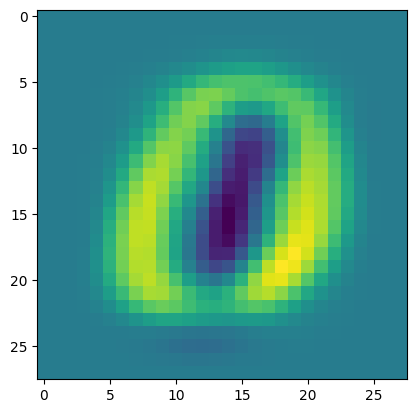In :
imshow(reshape(ESigma.vectors[:, 2], (28,28))');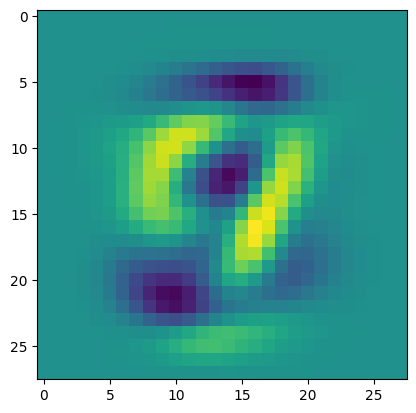In :
imshow(reshape(ESigma.vectors[:, 3], (28,28))');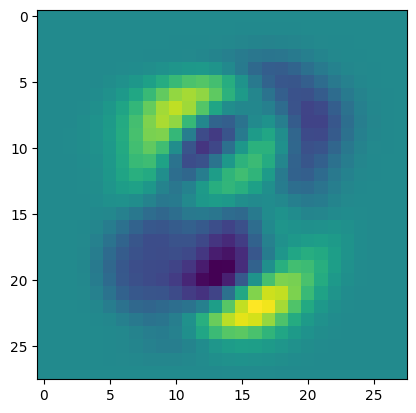In :
imshow(reshape(ESigma.vectors[:, 4], (28,28))');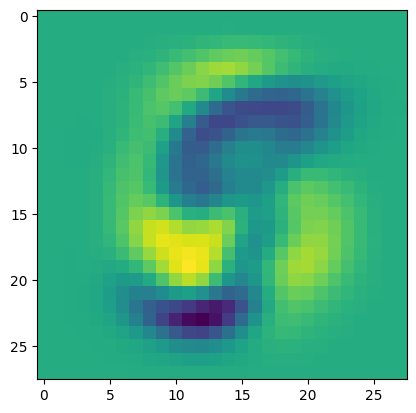In :
imshow(reshape(ESigma.vec
tors[:, 5], (28,28))');

syntax: missing comma or ) in argument list

Stacktrace:
 top-level scope at In:2
 include_string(::Function, ::Module, ::String, ::String) at ./loading.jl:1091
In :
plot(ESigma.values)
ylabel("eigenvalue")
xlabel("index of eigenvalue")
title("Eigenvalues of MNIST Covariance Matrix");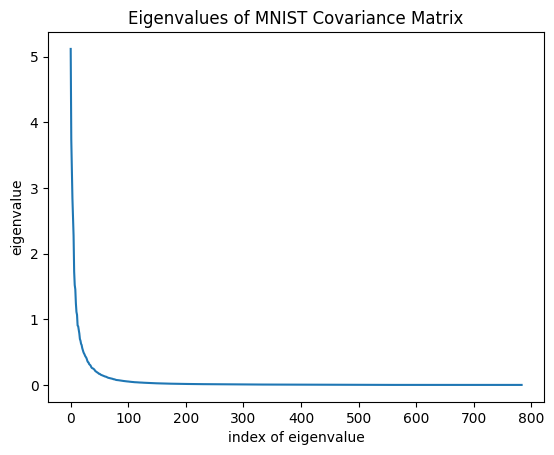PCA can represent objects in low dimension without losing information.

In :
D = 5;

In :
A = ESigma.vectors[:, 1:D]';

In :
# original image
i = 1337;
imshow(reshape(train_x[:,i], (28,28))');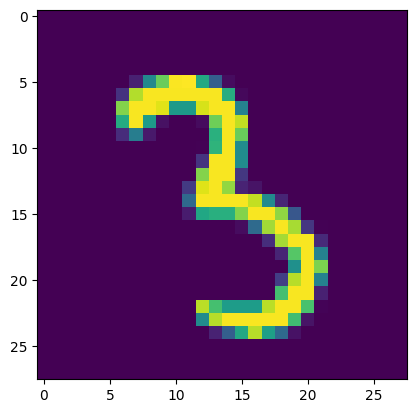In :
# dimension-reduced image
x_dr = A * train_x_minus_mean[:,i]
x_recovered = A' * x_dr + train_x_mean;

# original image
imshow(reshape(x_recovered, (28,28))');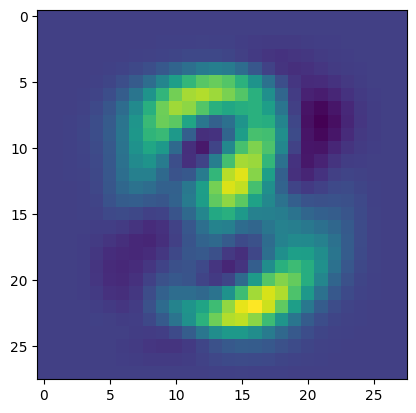Still enough to classify the image!

In [ ]:


In :
Matrix(X)

UndefVarError: X not defined

Stacktrace:
 top-level scope at In:1
 include_string(::Function, ::Module, ::String, ::String) at ./loading.jl:1091

## Sparsity¶

In :
n = 4096;
X = sprand(n,n,0.1);
Y = sprand(n,n,0.1);

In :
# visualize part of it
imshow(X[1:64,1:64]);
colorbar();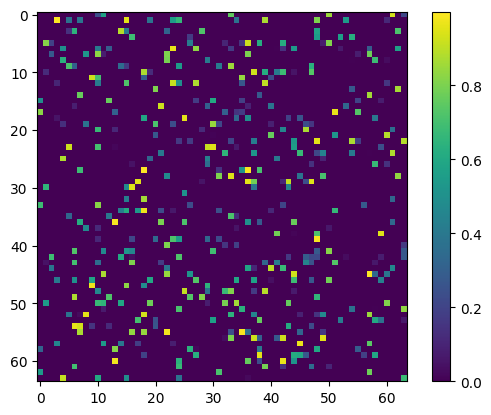In :
# get the size of X in bytes
Base.summarysize(X)

Out:
26896696
In :
# type of X
typeof(X)

Out:
SparseMatrixCSC{Float64,Int64}
In :
Xdense = Matrix(X);
Ydense = Matrix(Y);
typeof(Xdense)

Out:
Array{Float64,2}
In :
Base.summarysize(Xdense)

Out:
134217768
In :
# what's the ratio?
Base.summarysize(X) / Base.summarysize(Xdense)

Out:
0.20039594161631416
In :
# what fraction of X's entries are nonzero?
nnz(X) / length(X)

Out:
0.10007530450820923

Why are these numbers not equal?

In :
@time X * Y;

  1.312289 seconds (110.34 k allocations: 287.229 MiB, 0.31% gc time)

In :
dense_mmpy_time = @elapsed Xdense * Ydense

Out:
1.174083337

What happened here???

In :
# make X and Y less dense
Xsparser = sprand(n,n,0.01);
Ysparser = sprand(n,n,0.01);
@time Xsparser * Ysparser;

  0.121884 seconds (8 allocations: 94.578 MiB)

In :
densities = [0.001, 0.003, 0.01, 0.03, 0.1, 0.3];
sparse_mm_times = Float64[];
for d in densities
Xsparse = sprand(n,n,d);
Ysparse = sprand(n,n,d);
push!(sparse_mm_times, @elapsed(Xsparse * Ysparse));
end

In :
loglog(densities, sparse_mm_times; label="sparse mmpy");
loglog(densities, densities * 0 .+ dense_mmpy_time; label="dense mmpy");
xlabel("density");
ylabel("matrix multiply runtime");
legend();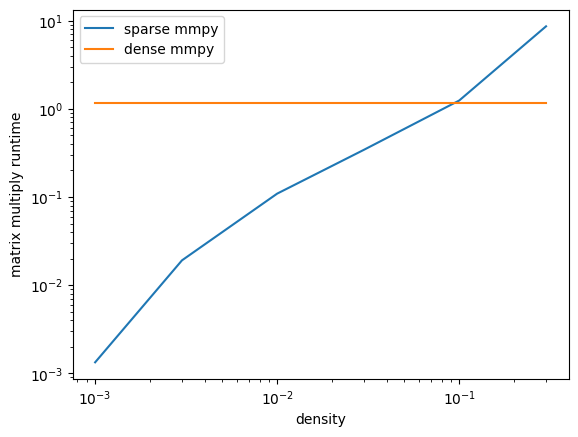In :
spv = X[:,1]

Out:
4096-element SparseVector{Float64,Int64} with 427 stored entries:
[16  ]  =  0.494497
[18  ]  =  0.826087
[34  ]  =  0.687849
[59  ]  =  0.318373
[63  ]  =  0.543537
[73  ]  =  0.460325
[75  ]  =  0.0852525
[90  ]  =  0.141923
[108 ]  =  0.0701148
[116 ]  =  0.994332
⋮
  =  0.570289
  =  0.54624
  =  0.202171
  =  0.403688
  =  0.420507
  =  0.538902
  =  0.265724
  =  0.191174
  =  0.67434
  =  0.384076
  =  0.188717
In :
spv.n

Out:
4096
In :
spv.nzind

Out:
427-element Array{Int64,1}:
16
18
34
59
63
73
75
90
108
116
125
127
134
⋮
4021
4029
4035
4040
4042
4062
4066
4068
4072
4080
4084
4089
In :
spv.nzval

Out:
427-element Array{Float64,1}:
0.4944967485040994
0.8260867791524058
0.6878492561841358
0.31837265760532185
0.5435373410214652
0.4603252124070474
0.08525252863099708
0.14192308123381947
0.07011482669433211
0.994332364738097
0.07054927165346192
0.3335951909204913
0.9303865975954675
⋮
0.2442214611309792
0.5702885838128535
0.5462397434084996
0.20217057750630207
0.40368809673279826
0.420507447700027
0.5389015609303036
0.2657240323430008
0.19117367805893304
0.674340219210197
0.3840757164610593
0.18871747643515846
In [ ]: